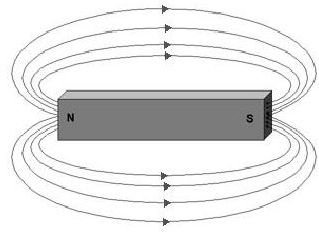## The Magnetic Field

Before going on to understand a magnetic circuit we must first understand what is meant by a magnetic field. Now you surely know that if you keep a magnet on the table and place a small iron object near it, it simply goes and sticks to the magnet. The force with which the piece is pulled decreases as its distance from the magnet increases. This phenomenon can be explained if you consider that the space around the magnet is permeated with a type of force which influences the iron piece near it. This force field is known as the magnetic field which can be visualized to be in the form of magnetic lines of flux. These are just imagine lines and do not exist actually but can only be experienced. The figure below shows these imaginary lines of magnetic flux surrounding a magnet.

## Lines of Magnetic Force## Features of Lines of Flux

There are several characteristics of lines of force which are as follows.

Direction: any line of flux always points towards the north seeking pole of a magnet provided it is not under the influence of another magnetic medium except the magnetic field of the earth.

Closed Line: all lines of flux form a closed loop which emerge from the North Pole into the South Pole of the magnet

Independent Region: lines of flux never intersect each other.

Repulsion: lines of flux which are in the same direction always repel each other.

## The Magnetic Circuit

The above discussion about magnetic field and lines of flux now leads us to the definition of a magnetic circuit. A magnetic circuit can be described as a complete closed path followed by any group of lines of magnetic flux. A magnetic circuit is quite similar to an electrical circuit in terms of various parameters which are associated with the magnetic circuit, some of which are described as follows.

Magneto-motive Force: just as electromotive force or emf makes electric current flow, similarly magneto-motive force or mmf makes the magnetic flux possible in a magnetic circuit. The units of mmf are ampere-turns, where turns signify the number of electric coil turns which is generating that mmf. Since number of terms is a dimensionless quantity, effectively the units of mmf are same as that of current, namely amperes.

Magnetic Field Strength: it refers to the mmf that exists per unit length of a magnetic circuit provided the circuit is homogeneous and of a uniform cross sectional area. In the earlier days it was also known as magnetizing force but this term is obsolete now. The units of magnetic field strength are amperes/meter.